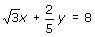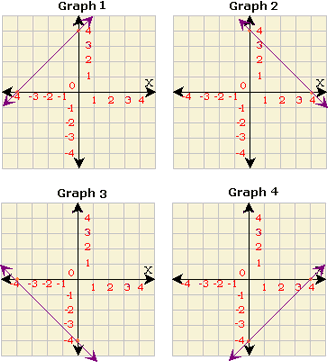## Definition Of Linear Equation

Linear equation is an equation of the form Ax + By = C, where A ≠ 0 and B ≠ 0. The graph of a linear equation is a straight line.

### Example of Linear Equation

The following are examples of linear equations.
5x + 7y = 1, - 13x + 6y = 0.5,### Solved Example on Linear Equation

#### Ques: Identify the graph that represents a linear function.A. Graph 1
B. Graph 2
C. Graph 3
D. Graph 4